# Review of Atomic Orbitals for Organic Chemistry

Last updated: November 22nd, 2019 |

General Chemistry Review: Atomic Orbitals for Organic Chemistry

[Note: the following article is intended as a quick review on atomic orbitals for students enrolled in an introductory organic chemistry class who are assumed to have taken general chemistry. It is by no means a complete introduction to atomic orbitals. Also: this post was co-authored with Matt Pierce of Organic Chemistry Solutions.  Ask Matt about scheduling an online tutoring session here. ]

## 1. The Most Important Graph Ever Made?Quote:

“If, in some cataclysm, all of scientific knowledge were to be destroyed, and only one sentence passed on to the next generation of creatures, what statement would contain the most information in the fewest words? I believe it is the atomic hypothesis that all things are made of atoms — little particles that move around in perpetual motion, attracting each other when they are a little distance apart, but repelling upon being squeezed into one another. In that one sentence, you will see, there is an enormous amount of information about the world, if just a little imagination and thinking are applied.”

–Richard P. Feynman

Well of course a chemist like myself is going to love a quote that extolls the central importance of chemistry to scientific knowledge.  You can always trust a barber to find an uplifting quote about the importance of haircuts.

That caveat aside, if one had to name a single graph which could be saved from a cataclysm for the next generation of creatures, my vote would be for this one: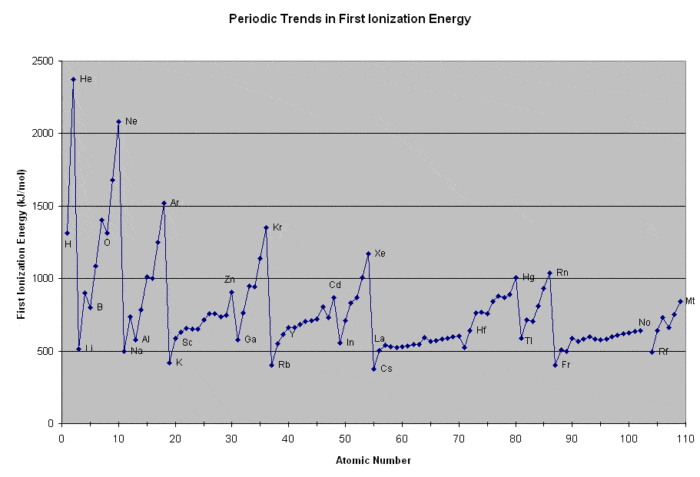What’s going on here?

• The x-axis shows every element of the periodic table, in increasing order of  atomic number.
• The y-axis shows the amount of energy required to ionize each neutral element to a charge of +1 (the “first ionization energy”).  In other words: “how easy is it to pull off an electron off each element?”.

There is a tremendous amount of information about atomic structure embedded in this extremely simple plot, but the concept itself isn’t so hard to understand: “how much energy does it take to rip an electron off a neutral element?”

Two important things to note:

• First, note the broad trend: generally, as elements increase in size, the amount of energy required to pull an electron away from the atom decreases. We can draw an analogy here to planets orbiting the sun: all else being equal, the further away a planet (electron) is from the sun (nucleus), the less attractive force there will be between the two (as measured by Newton’s law of gravitation in one case, and Coulomb’s law in the other). Indeed that “planetary” analogy was the basis for the Bohr model of the atom.
• Second, note the periodic trend: there are certain elements (He, Ne, Ar, Kr, Xe) which have unusually high ionization energies, followed by elements (Li, Na, K, Rb, and Cs) with unusually low ionization energies.

## 2. The Graph Is Explained By The Periodic Filling of Electronic Energy Levels (aka “Orbitals”)

Note how interpreting the diagram above flows naturally into a discussion about orbitals.

It was Bohr who first made the connection (1923) that the periodicity of atomic properties could be explained by the periodic filling of electronic energy levels.

In an early model of the atom (1913), it was imagined that electrons occupied progressively farther orbits around the nucleus, much like the planets in their ever-larger celestial spheres around the sun. While the “electronic energy levels” that Bohr described are indeed now called “orbitals”, the analogy ends there.  Bohr didn’t anticipate just how weirdly-behaved electrons can be, relative to planets. Nor did anyone else in 1913. [Note 1]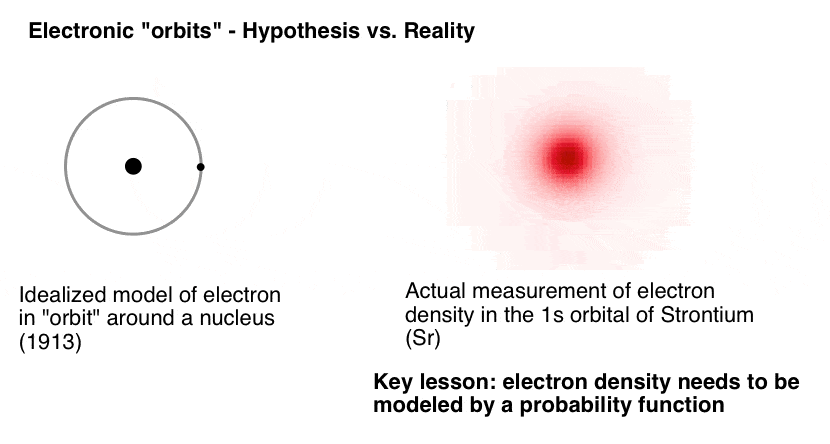What do I mean by “weirdly-behaved”? Well, if one knows the position and momentum of Venus, for example, then one can then calculate its positions at distant future times  (such as the transit of Venus predicted for 3:48 AM UTC on June 10, 2498).

Heisenberg showed that at the subatomic scale, there are limits to the precision with which one can know both the position and momentum of a particle like an electron. The result is that our knowledge of the exact positions of electrons are fuzzy;  they have to be described as probability functions. What we call “orbitals” are in fact  3-dimensional shapes where an electron with a given set of quantum numbers has a 95% chance of being found. [Note 2]

## 3. Orbitals Are Defined By The Three Quantum Numbers n, l, and m

These orbitals have properties which are defined by the three essential terms in a particular form of the Schroedinger wave equation:

• the principal quantum number n (1, 2, 3…), which is sometimes referred to as the “electron shell“, as it broadly relates to distance from the nucleus.
• (called the azimuthal quantum number, but knowing that name is not essential). For a given value of n,  the possible values of  ℓ can range from 0 up to (n–1). So when n = 1, ℓ = 0. When n = 2, ℓ can have values of 1 or 0.
The value of ℓ determines the shape of the orbital. For ℓ = 0, the shape of the orbital is spherical – an orbital we refer to as an s orbital.For ℓ = 1 (which is only possible when n = 2 or above) the orbital has a dumbbell-like shape, which we refer to as a p-orbital. The higher observed values are ℓ = 2 (d orbitals) and ℓ = 3 (f orbitals) which are themselves fascinating, but in true organic chemistry fashion, we will skip.
• m, which is the magnetic quantum number. The value of m depends on the value of ℓ, and can take the values –ℓ to +ℓ, inclusive of zero. So for ℓ = 1 (the p-orbital), m can have a value of –1, 0, or +1. The physical interpretation of this is the three orientations possible for the p-orbital, along the x, y, and z axes. m can take on five values for the d-orbitals (ℓ = 2) and seven values for the f orbitals (ℓ =3).

In addition to n, ℓ, and m, there is also fourth quantum number known as electronic spin, which can take on the value +½ or –½ for electrons.

Finally, the last bullet point:

•  No two electrons can have the same set of quantum numbers (which is to say, they cannot have the same quantum state) as elucidated in Wolfgang Pauli’s exclusion principle. (Electrons belong to a family of particles called fermions which share a property analogous to “one seatbelt per occupant”).  It’s an atom, not a clown car!What this means is that electrons in atoms “build up” in energy level in a well-defined pattern, starting with 1s (which can hold two electrons, with opposite spins), followed by 2s (which also holds two electrons) and then 2p (which due to the three possible values of m, can hold 3 × 2 = 6 electrons, and then 3s  (2 electrons) and so on.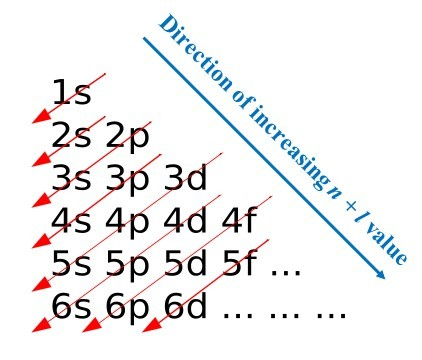## 4. A Tour Of The Electronic Configurations of The First 11 Elements

We could go on filling up electrons, but that’s not the intended point of todays post, which is merely to review the atomic configurations of each of the first 11 elements while referring back to their first ionization energies as a guide.

So let’s tour the electronic configurations of the first 11 elements, shall we?

## 5. The 1s shell: Hydrogen and Helium

Let’s look at the (first) ionization energies for hydrogen and helium:

Hydrogen: 1312 kJ/mol
Helium: 2372 kJ/mol

Hydrogen has a single electron in the 1s orbital with spin of either +1/2 or – 1/2 (they are of the exact same energy, except in a strong magnetic field).

We can represent the electronic configuration in two ways:

• the notation 1s1 , where 1 refers to the shell number (principal quantum number n), is the shape of the orbital (other values are p, d, and f) and the superscript refers to the number of electrons in that orbital
•  alternatively, with a box showing the orientation of electrons in each orbital (the direction of the arrow noting the spin). For a half-filled orbital, the direction of the spin is arbitrary; I’m going to draw it as “up” here and in all future examples, but it’s not incorrect to draw it as pointing down instead.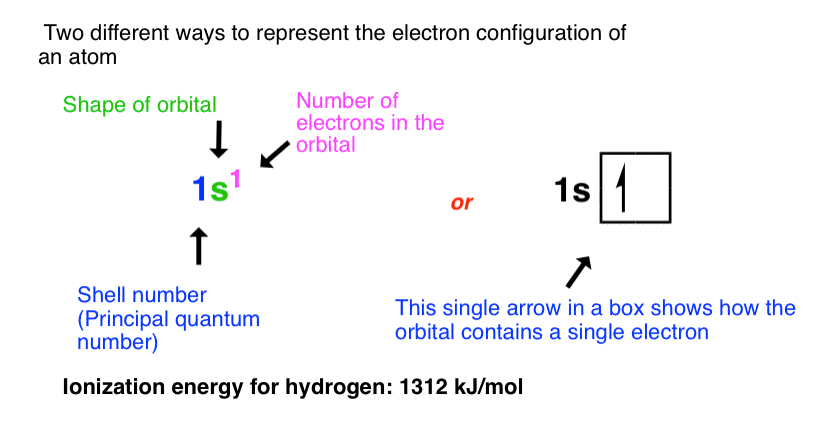Helium has two electrons in the 1s orbital, each with opposing spins (+1/2 and –1/2), which we represent below.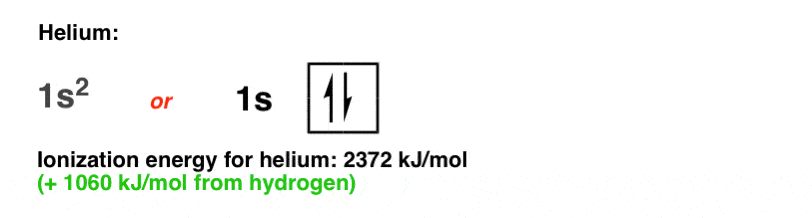It’s almost twice as difficult to remove an electron from helium than from hydrogen (compare: 2372 kJ/mol versus 1312 kJ/mol).

Why the higher ionization energy? Because each electron in the 1s orbital of helium now has two protons from the nucleus pulling on it instead of just one, which results in a stronger attractive force according to the Coulomb equation.

Note that it’s not exactly twice as much; we can rationalize the lower value as being due to repulsion between the two electrons in the 1s orbital.

## 6. The 2s Shell: Lithium and Beryllium

With the 1s orbital full, the third element (lithium)  must therefore have its electron placed in the 2s orbital, which is not only farther away from the nucleus, but also has the attractive force of the nucleus partially shielded by the intervening pair of electrons in the 1s orbital. This shielding leads the electron in the 2s orbital of lithium to only “feel” an effective nuclear charge of (3 – 2) = +1 from the nucleus.

Hence, this electron is particularly easy to rip off, requiring a mere 520 kJ/mol (compare to 2372 kJ/mol for helium!). Lithium metal will readily participate in chemical reactions; it will react slowly with water, for example.  Helium, on the other hand, has never been observed to combine with another element.

In contrast, the two electrons in the 1s shell of lithium never participate in reactions. We can call these chemically inert electrons the “inner shell” electrons, as opposed to the “outer shell” , or “valence” electron in the 2s orbital.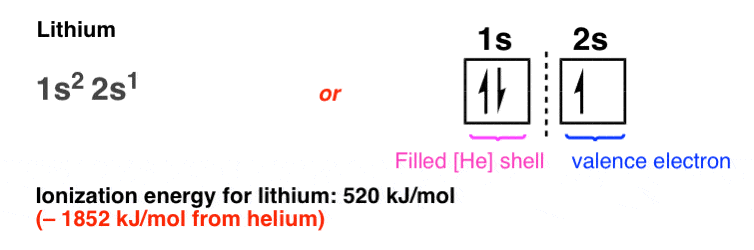Since all the interesting chemistry happens with the electrons in the 2s orbital (and not the electrons in the “inert”, closed, 1s shell) a useful shorthand is to draw the electronic configuration of lithium as [He] 2s1 , meaning that lithium has the electronic configuration of helium, plus a single valence electron in the 2s orbital.

In the fourth element, beryllium, the 2s orbital becomes fully occupied with a pair of electrons of opposite spin. Note the increased ionization energy (+379 kJ/mol from lithium) as the electrons feel a greater attraction from the extra proton in the nucleus.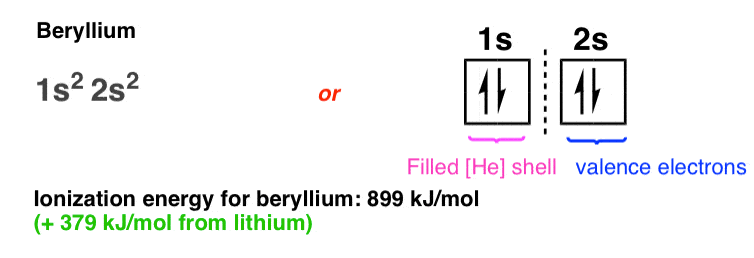Also note that although beryllium has a filled 2s orbital,  in no way does it behave like a noble gas. Beryllium metal reacts readily with oxygen to form an oxide layer, for example.  Unlike the gap in energy between 1s and 2s, the gap in energy between the 2s orbital and the next-highest energy level (2p) orbital is relatively small, such that these orbitals, when filled, can together hold a combined “octet” of valence electrons.

## 7. The 2p Shell: Boron, Carbon, and Nitrogen

With the 2s now full, additional electrons must now be placed in an even higher energy level, the 2p orbital.

Unlike the s orbitals, which can only hold two electrons apiece, each set of p orbitals can hold six. Our interpretation of this is that each level of p orbitals (2p, 3p, 4p, etc.) is comprised of three individual dumbbell-shaped p orbitals that are aligned at right angles to each other along the x, y, and z axes.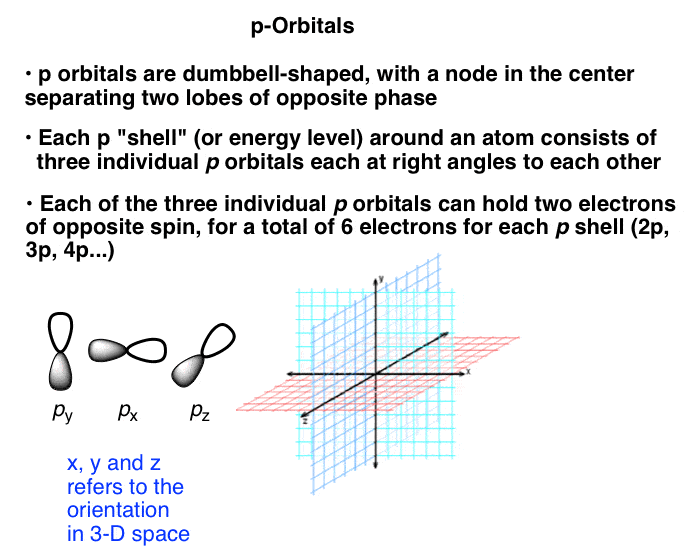Here’s what the electronic configuration looks like for boron. (Note: we could also choose to label the three 2p orbitals as 2px, 2py, and 2pz, but for our purposes all of these orbitals are of equivalent energies and it serves no purpose to do so here).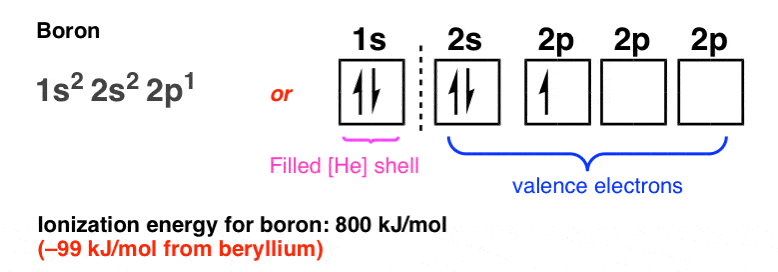Note how the ionization energy of boron is slightly lower (99 kJ/mol lower) than that of beryllium, from which we can interpret that the 2p orbital is slightly farther away from the nucleus than the 2s orbital. [Note 3].

The ionization energy progresses sharply upwards from boron to carbon…. (+ 286 kJ/mol)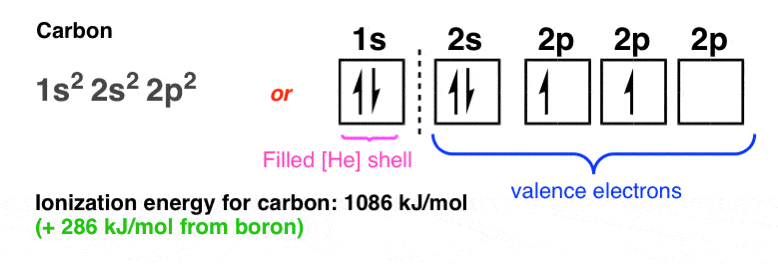… and then from carbon to nitrogen (+316 kJ/mol):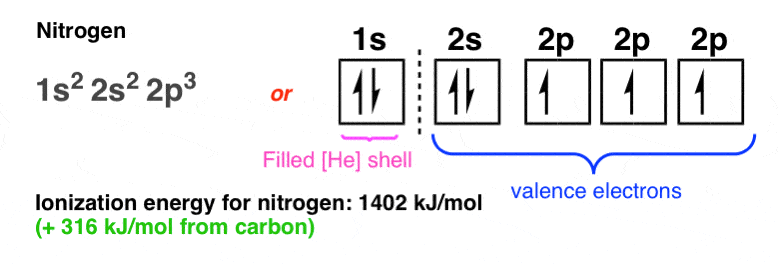## 8. A Sudden Dip At Oxygen

Then, at oxygen, there’s a sudden dip in the ionization energy (–89 kJ/mol):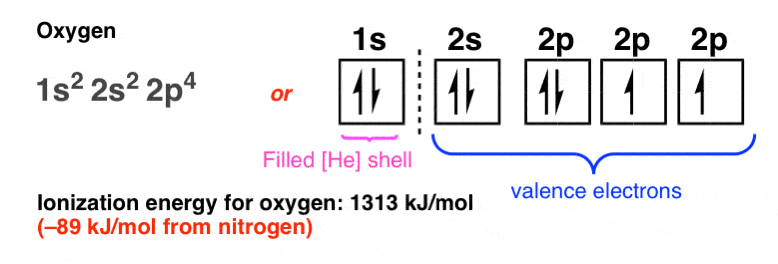Why?

Ever got on a bus to find out there are no remaining empty seats, and you have to (horrors!) sit next to a total stranger?

That’s essentially what’s happening here: with each of the 2p orbitals (px, py, pz) singly occupied, any additional electrons will have to pair up. That dip in ionization energy reflects greater electron-electron repulsion in a doubly occupied orbital, making the electron (slightly) easier to pull off.

## 9. The Electronic Configuration Of Fluorine

From oxygen to fluorine the ionization energy climbs again (+368 kJ/mol from oxygen):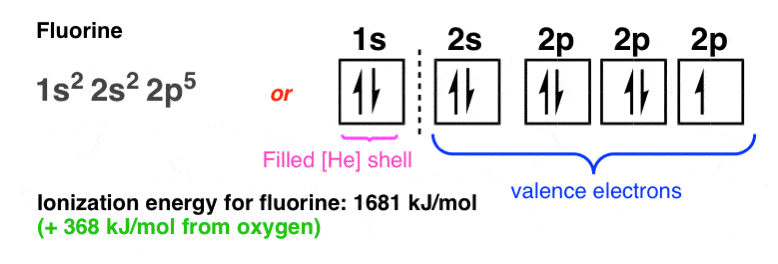## 10. A Maximum At Neon

And then, at neon we finally reach another local maximum of ionization energy (+399 kJ/mol from fluorine):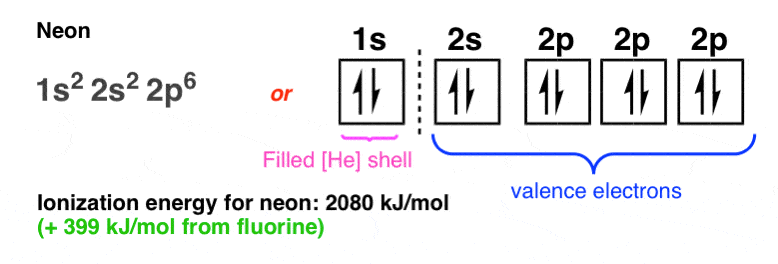Here we have reached the maximum capacity of the 2s and 2p orbitals (acting together as an “octet”), where each electron in the octet will “feel” the maximum effective charge from the nucleus. In neon, each of the eight electrons in the valence “octet” itself feels a net force of +8 from the nucleus (ten protons in the nucleus minus two electrons in the intervening 1s shell)  and a certain amount of electron-electron repulsion in the filled 2s and 2p orbitals.

Neon is a local maximum where the Coulombic attractive term is maximized, and so is ionization energy.  This is what gives rise to the familar “octet rule”, where atoms are said to “seek out full octets” and so on.

## 11. The 3s orbital: Sodium

Let’s just go one atom further to illustrate the dramatic difference in behaviour between sodium and neon.

There’s a big gap between the energies of the orbitals in the n=2 shell (2s and 2p) and the orbitals in the n=3 shell (3s and 3p).

The 3s orbital is farther away from the nucleus and the attractive force from the nucleus is shielded by the 10 electrons in lower-energy orbitals. We say that the “effective nuclear charge” felt by an electron in the sodium 3s orbital is just 1. (i.e. 11 – 10 = 1).

The ionization energy of sodium is a meagre 496 kJ/mol, which is 1584 kJ/mol less than neon. Sodium gives up its valence electron readily, reacting even with water (violently!); in contrast, no other element has ever been observed to combine with neon.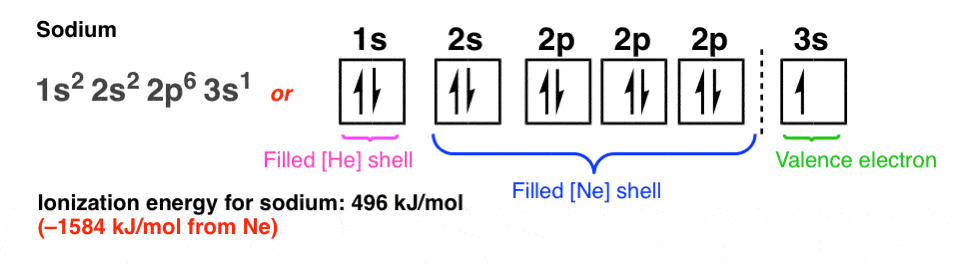We’re going to stop at this point, but you can imagine where it goes from here. [Note 4]

## 12. Endnote: A Puzzle. What About A Simple Molecule Like CH4?

So here’s a question. If the atomic configuration of carbon is [He]2s22p2, and the atomic configuration of hydrogen is 1s1, what might we predict the structure of the simplest hydrocarbon (CH4) to look like?

• Wouldn’t we expect to see C-H bonds along the px, py, and pz axes (bond angles of 90°) and then a fourth C-H bond in the 2s orbital as far away from the other electrons as possible (135° maybe) ?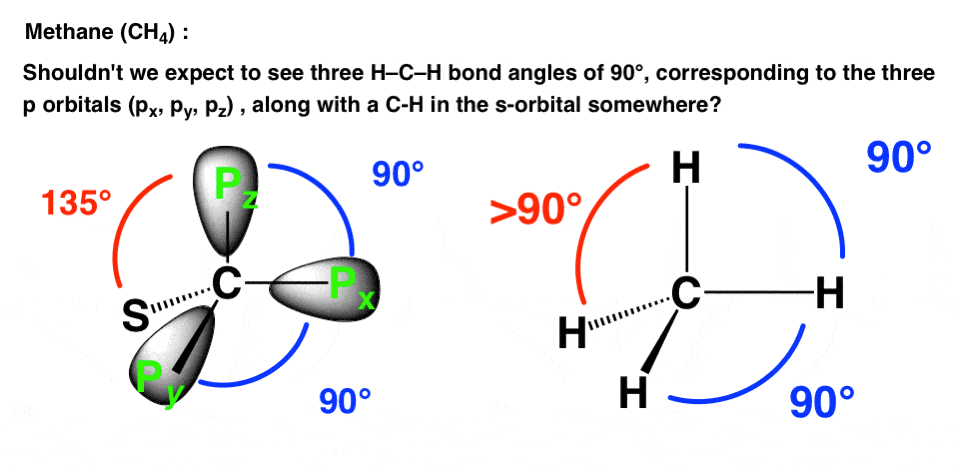• Wouldn’t we predict different bond lengths for the C-H bonds attached to p orbitals (farther away from nucleus) than for the C-H bond attached to the s-orbital?
• Wouldn’t we expect a (small) dipole moment for CH?

• All the C-H bonds have identical bond lengths (1.09 Angstrom).
• All C–H bond angles are identical. The hydrogens are arranged around carbon in a perfect tetrahedron, with all H-C-H bond angles being 109.5°.
• There is no dipole moment.

What gives? How can this possibly be true, given what we now know about the s and p orbitals? How can we explain this situation where the distinctions between the 2s and 2p orbitals have been completely wiped out?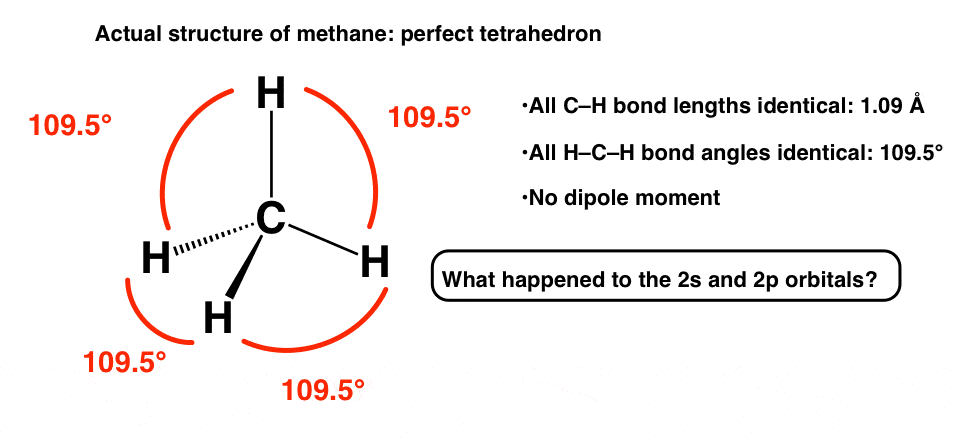So what’s going on? We’ll talk about that in the next post.

## Next Post: Why Is Methane Tetrahedral?

Thanks again to Matt for helping with this post. Hire Matt as your tutor!

## Comment section

### 3 thoughts on “Review of Atomic Orbitals for Organic Chemistry”

1.Brooster says:

This material leaves me with more questions than answers, which I feel is a good thing. One idea I do have, is the potential that an S orbital although shown to be a sphere, could have its electrons spinning in elaborate waves but always to be found within the sphere as stated above. The reason I state this is the view that wave/partical duality seems like a given, and what blows this bunnies brain, is the node is a non existent position that all electrons have, but can never be found there. Its almost as if the particle is flickering in and out of existence. Apparently this has been speculated before, but was something I felt myself must be the case. It gives rise to it being a particle, behaving as a wave, till the node is approached, then it briefly disappears to come back into verification on the other side of the dumbbell. The only issue I have with these models, is the one question that I wished I had of asked or thought about early on. How can positively charged particles, aka protons, remain in the nucleus of an atom. Ive played with magnets, and simply put, the repulsion makes it seem like a false model. I am not a physicist, nor overtly educated, but something must be apposing the repulsion,Quantum gravity perhaps. If there is such a thing. Newtons laws keep asserting, but in the realm of quantum, maybe everything is far from the experienced.

1.James says:

Regarding protons, they are held together in the nucleus by the strong nuclear force, which operates over extremely short distances.

2.James Ashenhurst says:

Hi. Well, as Neils Bohr said, “Hvis man kan sætte sig ind i kvantemekanik uden at blive svimmel, har man ikke forstået noget af det” (If you can fathom quantum mechanics without getting dizzy, you don’t get it).

As for the nucleus, what keeps the positively charged protons together is the strong nuclear force, which only operates when nuclei are extremely close to each other. (Its strength tails off with distance to the 6th power).

This site uses Akismet to reduce spam. Learn how your comment data is processed.